# 金融工程第 1～3 章参考答案

## 第一次作业

### Exercise 1.3

【1.3】 In the one-period binomial model of Section 1.1, suppose we want to determine the price at time zero of the derivative security $V_1=S_1$ (i.e. the derivative security pays off the stock price.) (This can be regarded as a European call with strike price K = 0). What is the time-zero price $V_0$ given by the risk-neutral pricing formula(1.1.10)?
【Reference Answer】 Follow no-arbitrage condition, we have: $$V_0 = \frac{V_1}{(1+r)}$$

where $V_1=S_1$, so we can substitute $V_1$ as $S_1$:
$$V_0 = \frac{S_1}{(1+r)}$$

Under risk-neutral measure:
$$S_1 = \tilde{p} \times u \times S_0 + \tilde{q}\times d \times S_0$$where $\tilde{p} = \frac{1+r-d}{u-d}, \tilde{q} = 1-\tilde{p}$, u is up factor and d is down factor, r is no-risk interest rate. Then:
$$S_1 = \frac{1+r-d}{u-d} \times u \times S_0 + (1-\frac{1+r-d}{u-d})\times d \times S_0$$Simplied this equation:
$$S_1 = (1+r) \times S_0$$

So, $V_0 = S_0$. This is the time-zero price of the derivative security.

### Exercise 1.8

【1.8】 (Asian option). Consider the three-period model of Example 1.2.1, with $S_0=4, u = 2, d = \frac{1}{2}$, and take the interest rate $r = \frac{1}{4}$, so that $\tilde{p} = \tilde{q} = \frac{1}{2}$. For n = 0, 1, 2, 3, define $Y_n = \sum^n_{k=0}S_k$ to be the sum of the stock prices between times zero and n. Consider an Asian call option that expires at time three and has strike K=4 (i.e., whose payoff at time three is $(\frac{1}{4}Y_3 - 4)^+$). This is like a European call, except the payoff of the option is based on the average stock price rather than the final final stock price. Let $v_n(s, y)$ denote the price of this option at times n if $S_n = s$ and $Y_n = y$. In particular, $v_3(s, y) = (\frac{1}{4}y - 4)^+$.
(i) Develop an algorithm for computing $v_n$ recursively. In particular, write a formula for $v_n$ in terms of $v_{n+1}$.
(ii) Apply the algorithm developed in (i) to compute (4.4), the price of the Asian option at time zero.
(iii) Provide a formula for $\delta_n(s, y)$, the number of shares of stock that should be held by replicating portfolio at times n if $S_n=s$ and $Y_n = y$.

(i) Follow no-arbitrage condition, we have:

\begin{align} v_n & =\frac{1}{1+r}[\tilde{p}v_{n+1}(us)+\tilde{q}v_{n+1}(ds)] \\ & = \frac{1}{1+\frac{1}{4}}[\frac{1}{2}v_{n+1}(2s)+\frac{1}{2}v_{n+1}(\frac{1}{2}s)] \end{align}

So the formula for $v_n$ in terms of $v_{n+1}$ is:
$$v_n(s, y) = \frac{2}{5}[v_{n+1}(2s, y +2s) + v_{n+1}(\frac{1}{2}s, y +\frac{1}{2}s)]$$

(ii) Following figure presents the path of stock price and the asian option’ s payoff at time three.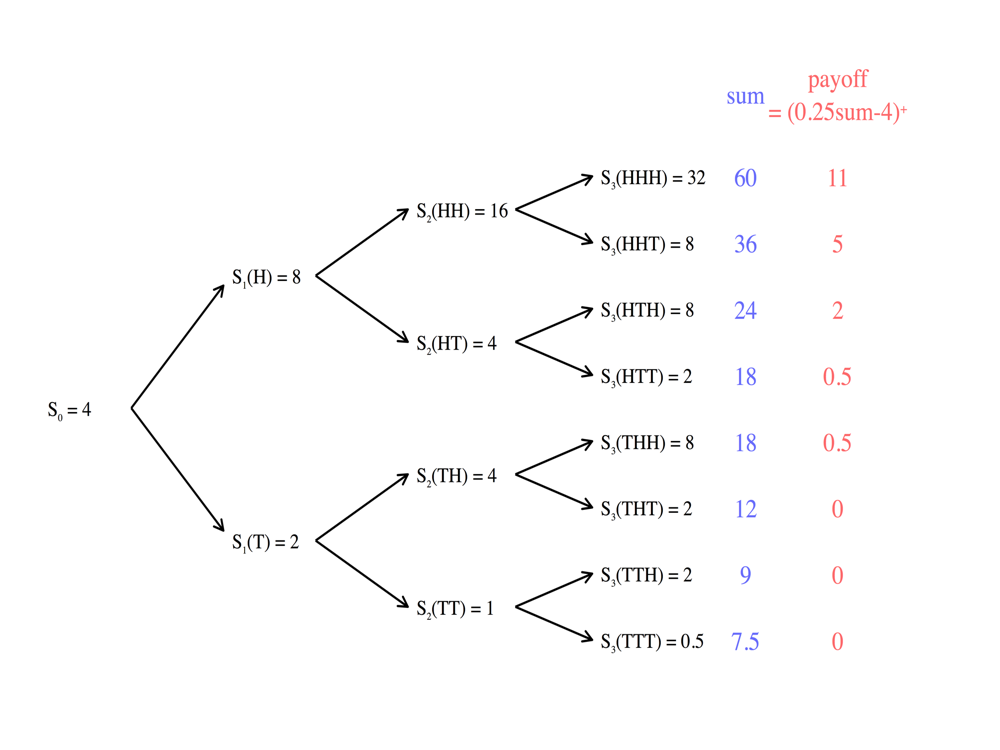Then we can get:
$$v_2(HH) = \frac{1}{1+r}[\frac{1}{2}v_3(HHH)+\frac{1}{2}v_3(HHT)] = 6.4$$

$$v_2(HT) = \frac{1}{1+r}[\frac{1}{2}v_3(HTH)+\frac{1}{2}v_3(HTT)] = 1$$

$$v_2(TH) = \frac{1}{1+r}[\frac{1}{2}v_3(THH)+\frac{1}{2}v_3(THT)] = 0.2$$

$$v_2(TT) = \frac{1}{1+r}[\frac{1}{2}v_3(TTH)+\frac{1}{2}v_3(TTT)] = 0$$

Next, at time one, we have:$$v_1(H) = \frac{1}{1+r}[\frac{1}{2}v_2(HH)+\frac{1}{2}v_2(HT)] = 2.96$$

$$v_1(T) = \frac{1}{1+r}[\frac{1}{2}v_2(TH)+\frac{1}{2}v_2(TT)] = 0.08$$

Final, we back to time zero:$$v_0 = \frac{1}{1+r}[\frac{1}{2}v_1(H)+\frac{1}{2}v_1(T)] = 1.216$$

So, the price of the Asian option at time zero is 1.216.

(iii). Follow theorem (1.2.2)and equation (1.2.17), we can get:
$$\delta_n(s, y) = \frac{v_{n+1}(us, y + us) - v_{n + 1}(ds, y + ds)}{(u-d)s}$$

This is the formula we need to provide.

### 附加题：试证明 0 < d < 1+r < u 时，无套利成立

(i) 如果 d > 1+r，我们可以以无风险利率借入资金然后投资于股票。即使期末股价下跌，我们获得的收益率 d 依然大于无风险利率，即实现了无风险套利，这违背了无套利原则；
(ii) 如果 u < 1+r，我们可以买空股票然后将资金以无风险利率存入银行。即使期末股价上升，我们依然能够或得收益，即实现了无风险套利，这违背了无套利原则。

## 第一次小测

### Q1

【Q1】 详述期权 4 个基本操作策略，并指出其进场时机、最大损失、最大获利、进场成本、损益两平点。（30%）

• 买入看涨期权：1. 进场时机：预期股价大幅上涨；
2. 最大损失：权利金 M；
3. 最大获利：无限大；
4. 进场成本：权利金 M；
5. 损益平衡点：A 点，股价为 K+M 时。
• 卖出看涨期权：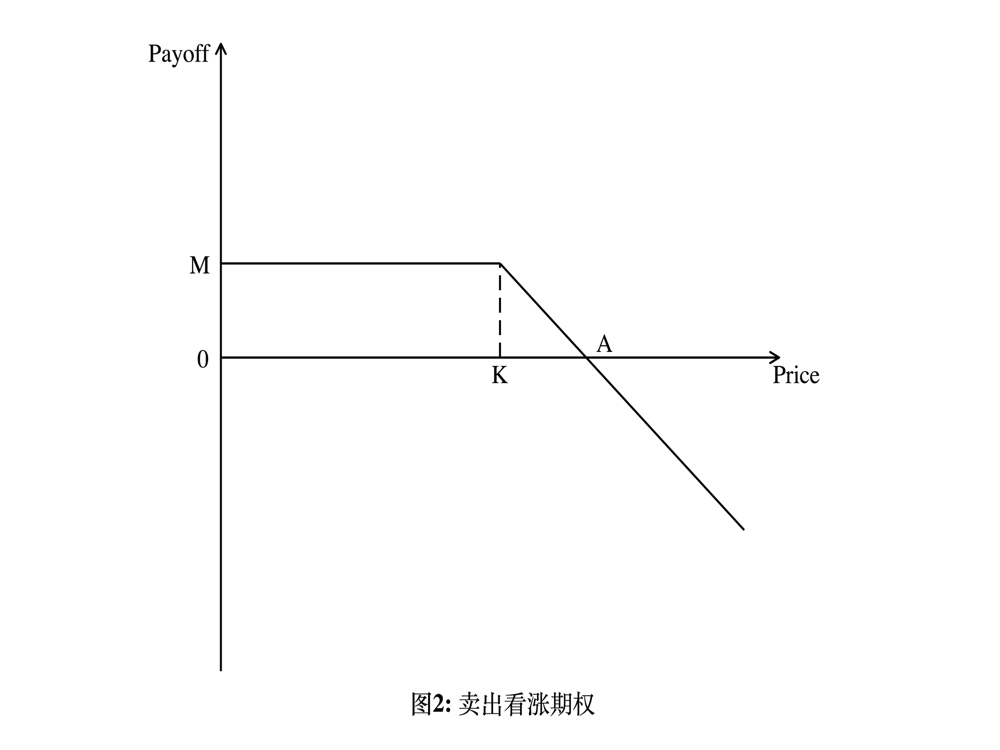1. 进场时机：预期股价下跌；
2. 最大损失：无限大；
3. 最大获利：权利金 M；
4. 进场成本：履约保证金；
5. 损益平衡点：A 点，股价为 K+M 时。
• 买入看跌期权：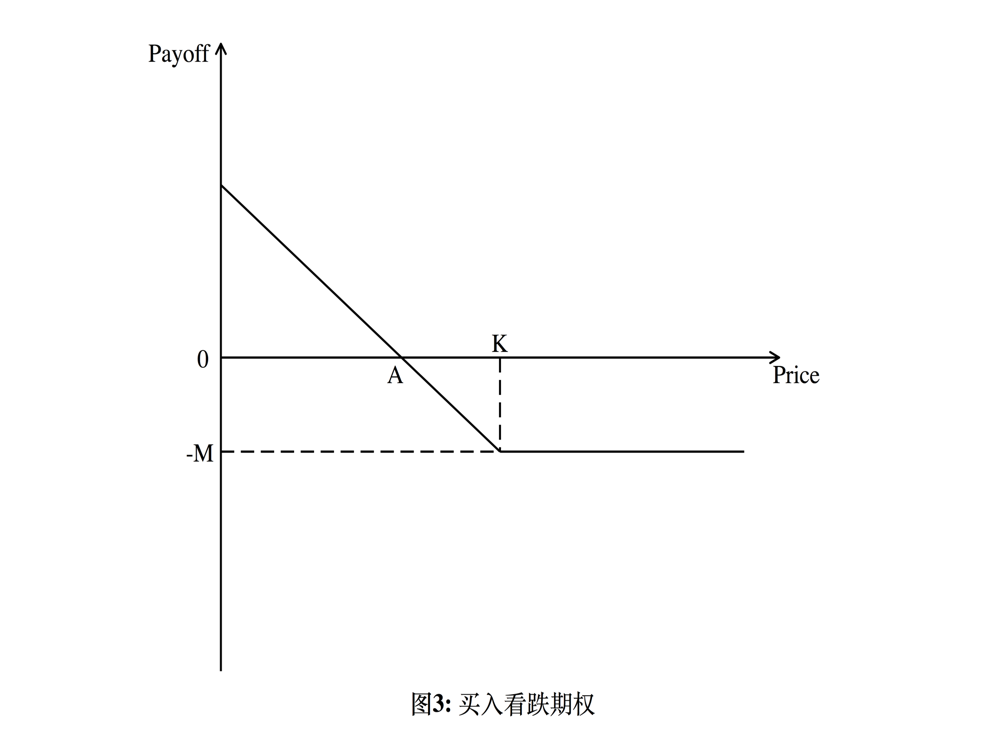1. 进场时机：预期股价下跌，但是担心股价上涨；
2. 最大损失：权利金 M；
3. 最大获利：股价为 0 时，最大获利为 K-M；
4. 进场成本：权利金 M；
5. 损益平衡点：A 点，股价为 K-M 时。
• 卖出看跌期权：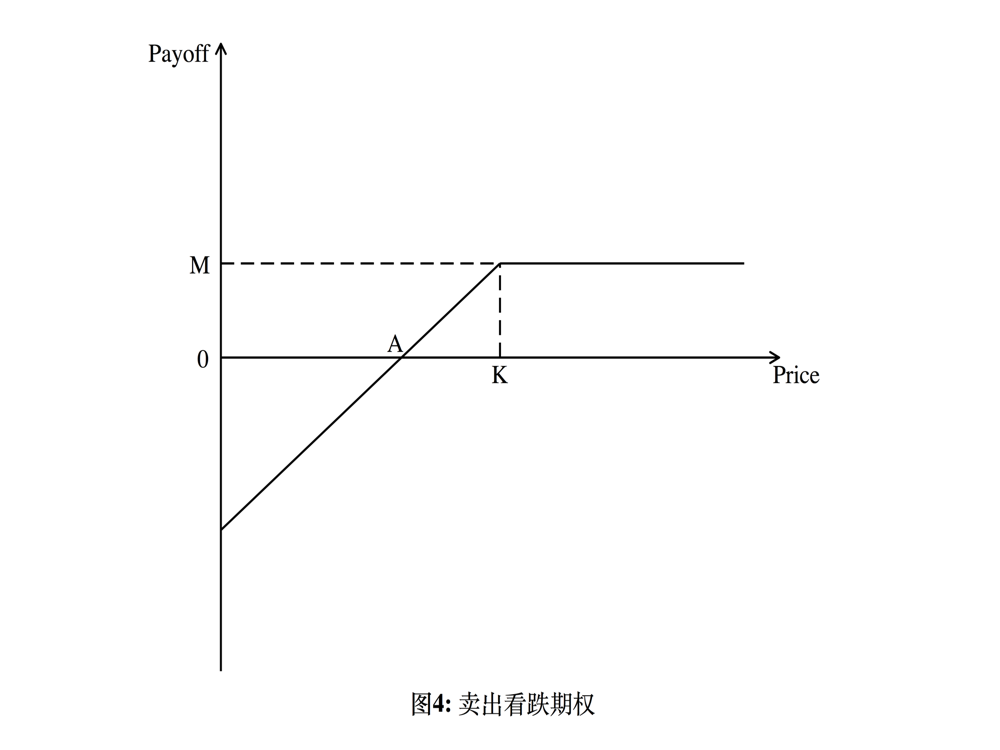1. 进场时机：预期股价上涨；
2. 最大损失：当股价为 0 时，最大损失为 K-M；
3. 最大获利：权利金 M；
4. 进场成本：履约保证金；
5. 损益平衡点：A 点，当股价为 K-M 时。

### Q2

【Q2】 试详述期权的价格类型。（20%）

1. 实值期权：看涨期权在执行价格低于标的价格时或看跌期权在执行价格高于标的价格时，具有内涵价值和可能在日后履行时获利的期权；
2. 平值期权：期权在执行价格等于标的价格时，立即执行不会获利或亏损的期权；
3. 虚值期权：看涨期权在执行价格高于标的价格时或看跌期权在执行价格低于标的价格时，不具有内涵价值的期权。

### Q3

【Q3】 试阐述如何形成一个投资组合来复制期权的价格（重点：说明无套利与风险中性的关系）。（25%）

$$X_0 = V_0$$

$$X_1 = \Delta_0S_1 + (1+r)(X_0-\Delta_0S_0)$$

\begin{align} X_1(H) &= \Delta_0S_1(H) + (1+r)(X_0-\Delta_0S_0) \\ &= \Delta_0(S_1(H)-(1+r)S_0) + (1+r)X_0 \end{align} \tag{3.1}

\begin{align} X_1(T) &= \Delta_0S_1(T) + (1+r)(X_0-\Delta_0S_0) \\ &= \Delta_0(S_1(T)-(1+r)S_0) + (1+r)X_0 \end{align} \tag{3.2}

$$X_1(H) = V_1(H) \tag{3.3}$$

$$X_1(T) = V_1(T) \tag{3.4}$$

$$X_0 + \Delta_0 \left( \frac{1}{1+r}S_1(H) - S_0 \right) = \frac{V_1(H)}{1+r} \tag{3.5}$$

$$X_0 + \Delta_0 \left( \frac{1}{1+r}S_1(T) - S_0 \right) = \frac{V_1(T)}{1+r} \tag{3.6}$$

$式(3.5) \times \tilde{p} + 式 (3.6) \times \tilde{q}$可得：

\begin{align} X_0 + \Delta_0 \left( \frac{1}{1+r}[\tilde{p}S_1(H) + \tilde{q}S_1(T)] - S_0 \right) \\ = \frac{1}{1+r}[\tilde{p}V_1(H) + \tilde{q}V_1(T)]\end{align} \tag{3.7}

$$S_0 = \frac{1}{1+r}[\tilde{p}S_1(H) + \tilde{q}S_1(T)] \tag{3.8}$$

$$X_0 = \frac{1}{1+r}[\tilde{p}V_1(H) + \tilde{q}V_1(T)] \tag{3.9}$$

$$V_0 = \frac{1}{1+r}[\tilde{p}V_1(H) + \tilde{q}V_1(T)] \tag{3.10}$$

$$S_0 = \frac{1}{1+r}[\tilde{p}uS_0 + (1-\tilde{p}dS_0)] = \frac{S_0}{1+r}[(u-d)\tilde{p}+d] \\ \tilde{p} = \frac{1+r-d}{u-d}, \qquad \tilde{q} = \frac{u - 1-r}{u-d}$$

### Q4

【Q4】 给定$S_0 = 4, u = 2, d = 0.5, r = 0.25$ , 考虑一回望期权到期报酬为$V_3 = S_T - min_{0\leq n \leq 3}S_n = S_T-m_3$，试利用风险中性评价法，找出该期权期初的无套利价格$V_0$。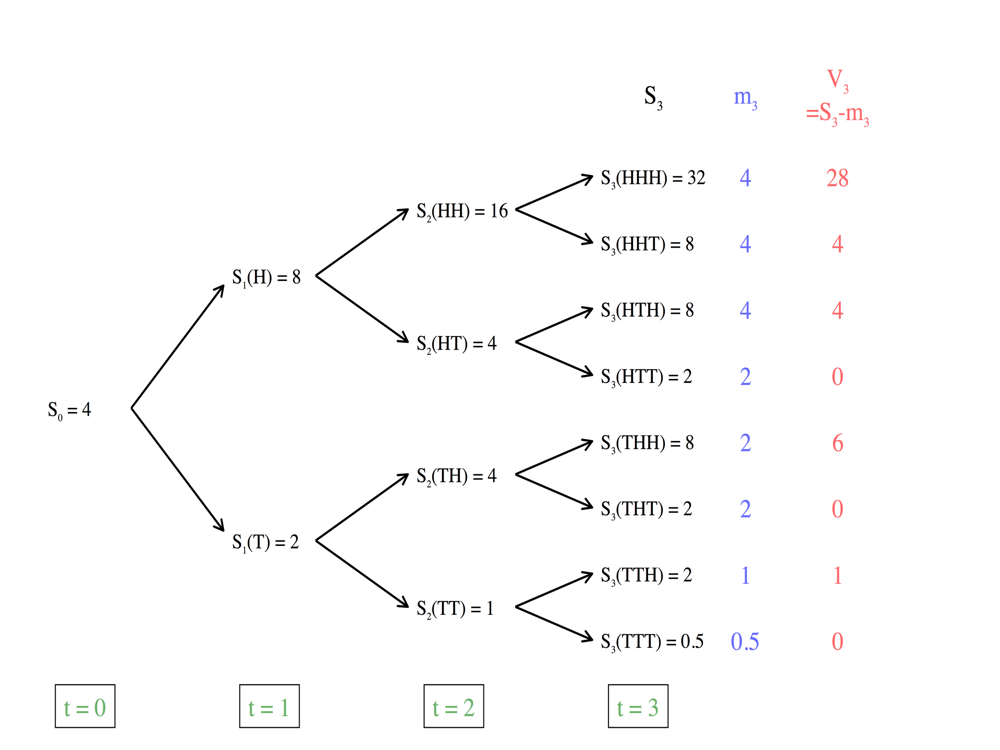$$\left\{ \begin{array}{lr} V_2(HH) = \frac{1}{1+r}[\frac{1}{2}V_3(HHH) + \frac{1}{2}V_3(HHT)] = 12.8 \\ V_2(HT) = \frac{1}{1+r}[\frac{1}{2}V_3(HTH) + \frac{1}{2}V_3(HTT)] = 1.6 \\ V_2(TH) = \frac{1}{1+r}[\frac{1}{2}V_3(THH) + \frac{1}{2}V_3(THT)] = 12.4 \\ V_2(TT) = \frac{1}{1+r}[\frac{1}{2}V_3(TTH) + \frac{1}{2}V_3(TTT)] = 0.4 \\ \end{array} \right.$$

$$\left\{ \begin{array} {lr} V_1(H) = \frac{1}{1+r}[\frac{1}{2}V_2(HH) + \frac{1}{2}V_2(HT)] = 5.76 \\ V_1(T) = \frac{1}{1+r}[\frac{1}{2}V_2(TH) + \frac{1}{2}V_2(TT)] = 1.12 \end{array} \right.$$

$$V_0 = \frac{1}{1+r}[\frac{1}{2}V_1(H) + \frac{1}{2}V_1(T)] = 2.752$$

## 第二次作业

### Exercise 2.4

【2.4】 Toss a coin repeatedly. Assume the probability of head on each toss is 0.5, as is the probability of tail. Let $X_j = 1$ if the $j$th toss results in a head and $X_j = -1$ if the $j$th toss results in a tail. Consider the stochastic process $M_0, M_1, \cdots$ define by $M_0$ and

$$M_n = \sum^n_{j = 1}X_j , n \geq 1$$

This is called a symmetric random walk; with each head, it steps up one, and with each tail, it steps down one.
(i) Using the properties of Theorem 2.3.2, show that $M_0, M_1, M_2, \cdots$ is a martingale.
(ii) Let $\sigma$ be a positive constant and, for $n \geq 0$, define

$$S_n = e^{\sigma M_n}\left(\frac{2}{e^\sigma + e^{-\sigma}}\right)^n$$

Show that $S_0, S_1, S_2, \cdots$ is a martingale. Note that even though the symmetric random walk $M_n$ has no tendency to grow, the “geometric symmetric random walk” $e^{\sigma M_n}$ have a tendency to grow, this is the result of putting a martingale into the (convex) exponential function (see Exercise 2.3). In order to again have a martingale, we must “discount” the geometric symmetric random walk, using the term $\frac{2}{e^\sigma + e^{-\sigma}}$ as the discount rate. This term is strictly less than one unless $\sigma = 0$.

(i)

\begin{align} E_n \left[ M_{n+1} \right] & = E_n \left[ \sum^{n+1}_{j=1} X_j \right] \\ & = E_n \left[ \sum_{j=1}^{n} + X_{n+1} \right] \\ & = E_n \left[ M_n + X_{n+1} \right] \\ & = M_n + E_n \left[ X_{n+1} \right] \\ & = M_n + \frac{1}{2} \cdot 1 + \frac{1}{2} \cdot \left( -1 \right) \\ & = M_n \end{align}

So, $M_0, M_1, M_2, \cdots$ is a martingale.

(ii)
\begin{align} E_n \left[ S_{n+1} \right] & = E_n\left[ e^{\sigma M_{n+1}} \left(\frac{2}{e^\sigma + e^{-\sigma}}\right)^{n+1} \right] \\ & = E_n \left[ e^{\sigma M_{n}} e^{\sigma X_{n+1}} \left( \frac{2}{e^\sigma + e^{-\sigma}} \right)^n \left( \frac{2}{e^\sigma + e^{-\sigma}} \right) \right] \\ & = S_n E_n\left[ e^{\sigma X_{n+1}} \left( \frac{2}{e^\sigma + e^{-\sigma}} \right) \right] \\ & = S_n \frac{2}{e^\sigma + e^{-\sigma}} E \left[ e^{\sigma X_{n+1}} \right] \\ & = S_n \cdot \frac{2}{e^\sigma + e^{-\sigma}} \cdot ( \frac{1}{2} \cdot e^\sigma + \frac{1}{2} \cdot e^{-\sigma}) \\ & = S_n \end{align}

So, $S_0, S_1, S_2, \cdots$ is a martingale.

### Exercise 2.5

【2.5】 Let $M_0, M_1, M_2, \cdots$ be the symmetric random walk of Exercise 2.4, and define $I_0 = 0$ and
$$I_n = \sum^{n-1}_{j = 0}M_j(M_{j+1}-M_j), n = 1, 2, \cdots$$
(i) Show that
$$I_n = \frac{1}{2}M^2_n - \frac{n}{2}$$

(ii) Let n be an arbitrary nonnegative integer, and let $f(i)$ be an arbitrary function of variable $i$, In terms of n and f, define another function $g(i)$ satisfying

$$E_n[f(I_{n+1})] = g(I_n)$$

Note that although the function $g(I_n)$ on the right-hand side of this equation may depend on n, the only random variable that may appear in its argument is $I_n$; the random variable $M_n$ may not appear. You will need to use the formula in part (i). The conclusion of part (ii) is that the process $I_0, I_1, I_2, \cdots$ is a Markov process.

(i)
\begin{align} 2I_n &= 2 \cdot \sum^{n-1}_{j=0}M_j(M_{j+1} - M_j) \\ & = 2 \cdot \sum^{n-1}_{j = 0}M_jM_{j+1} - 2 \cdot \sum_{j = 0}^{n-1}M_{j}^2 \\ & = 2 \sum_{j=0}^{n-1}M_jM_{j+1} + M^2_n - \sum_{j=0}^{n-1}M_{j+1}^2 - \sum_{j = 0}^{n-1}M_j^2 \\ & = M_n^2 - \sum_{j=0}^{n-1}(M_{j+1} - M_j)^2 \\ & = M_n^2 - \sum_{j=0}^{n-1}X_{j+1}^2 \\ & = M_n^2 - n \end{align}

So, $I_n = \frac{1}{2}M_n^2 - \frac{n}{2}$.

(ii)
First, we have
\begin{align} I_{n+1} &= \sum^{n}_{j = 0}M_j(M_{j+1}-M_j) \\ & = \sum^{n-1}_{j = 0}M_j(M_{j+1}-M_j) + M_n(M_{n+1} - M_n) \\ & = I_n + M_n(M_{n+1} - M_n) \end{align}

So, we can get
\begin{align} E_n[f(I_{n+1})] & = E_n[f( I_n + M_n(M_{n+1} - M_n) )] \\ & = E_n[f(I_n+M_nX_{n+1})] \\ & = \frac{1}{2}[f(I_n+M_n)+f(I_n-M_n)] \\ & = g(I_n) \end{align}

So, we can define $g(x) = \frac{1}{2}[f(x+\sqrt{2x+n}) + f(x - \sqrt{2x+n})]$, where $I_n = \frac{1}{2}M_n^2 - \frac{n}{2}$, $|M_n| = \sqrt{2I_n + n}$.

### Exercise 2.6

【2.6】 (Discrete-time stochastic integral). Suppose $M_0, M_1, \cdots$ is a martingale, and let $\Delta_0, \Delta_1, \cdots , \Delta_{N-1}$ be an adapted process. Define the discrete-time stochastic integral (sometime called a martingale transform) $I_0, I_1, \cdots, I_N$ by setting $I_0 = 0$ and
$$I_n = \sum^{n-1}_{j =0}\Delta_j(M_{j+1} - M_j), n = 1, \cdots, N$$ Show that $I_0, I_1, \cdots, I_N$ is a martingale.

Proof:
\begin{align} E_n[I_{n+1}] & = E_n \left[ \sum^{n}_{j=0}\Delta_j(M_{j+1}-M_j) \right] \\ & = E_n \left[ \sum^{n-1}_{j=0}\Delta_j(M_{j+1} - M_j) + \Delta_n(M_{n+1} - M_n) \right] \\ & = I_n + E_n\left[\Delta_n(M_{n+1} - M_n)\right] \\ & = I_n + \Delta_n \left[ E_n(M_{n+1}) - M_n \right] \\ & = I_n + 0 \\ & = I_n \end{align}
So, $I_0, I_1, \cdots, I_N$ is a martingale.

### Exercise 2.9

【2.9】 (Stochastic volatility, random interest rate). Consider a two-period stochastic volatility, random interest rate model of the type described in Exercise 1.9 of Chapter 1. The stock price and interest rates are shown in Figure 2.8.1.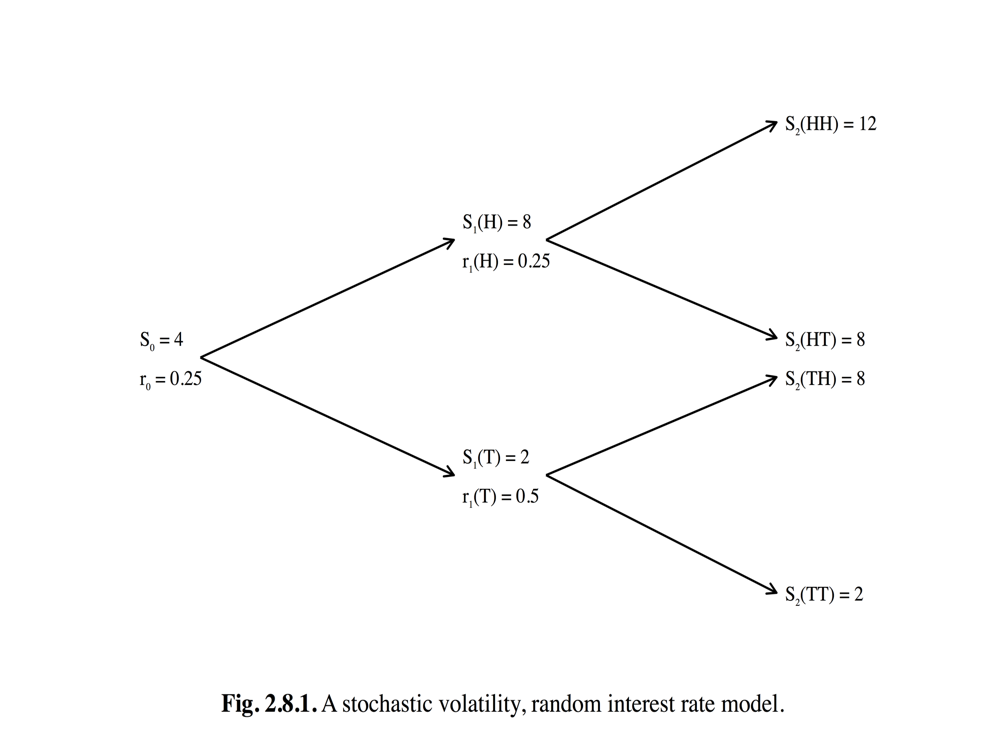(i) Determine risk-neutral probabilities

$$\tilde{P}(HH), \tilde{P}(HT), \tilde{P}(TH), \tilde{P}(TT),$$

such that the time-zero value of an option that pays off $V_2$ at time two is given by the risk-neutral pricing formula

$$V_0 = \tilde{E} \left[ \frac{V_2}{(1+r_0)(1+r_1)} \right]$$

(ii) Let $V_2 = (S_2 - 7)^+$. Compute $V_0, V_1(H)$, and $V_1(T)$.

(iii) Suppose an agent sells the option in (ii) for $V_0$ at time zero. Compute the position $\Delta_0$ she should take in the stock at time zero so that at time one, regardless of weather the first coin toss results in head or tail, the value of her portfolio is $V_1$.

(iv) Suppose in (iii) that the first coin toss results in head. What position $\Delta_1(H)$ should the agent now take in the stock to be sure that, regardless of weather the second coin toss results in head or tail, the value of her portfolio at time two will be $(S_2-7)^+$.

(i) From Fig.2.8.1 we can get:
\begin{align} u_0 &= \frac{S_1(H)}{S_0} = 2, \quad d_0 = \frac{S_1(T)}{S_0} = \frac{1}{2} \\ u_1(H) &= \frac{S_2(HH)}{S_1(H)} = 1.5, \quad d_1(H) = \frac{S_2(HT)}{S_1(H)} = 1 \\ u_1(T) &= \frac{S_2(TH)}{S_1(H)} = 4, \quad d_1(H) = \frac{S_2(TT)}{S_1(H)} = 1 \\ \end{align}
Then,
\begin{align} \tilde{p}_0 & = \frac{1+r_0-d_0}{u_0-d_0} = \frac{1}{2} , \quad \tilde{q} = \frac{1}{2} \\ \tilde{p}_1(H) &= \frac{1 + r_1(H) - d_1(H)}{u_1(H) - d_1(H)} =\frac{1}{2}, \quad \tilde{q}_1(H) = \frac{1}{2} \\ \tilde{p}_1(T) &= \frac{1 + r_1(T) - d_1(T)}{u_1(T) - d_1(T)} =\frac{1}{6}, \quad \tilde{q}_1(T) = \frac{5}{6} \\ \end{align}
So,
\begin{align} \tilde{p}(HH) & = \tilde{p}_0 \cdot \tilde{p}_1(H) = \frac{1}{4} \\ \tilde{p}(HT) & = \tilde{p}_0 \cdot \tilde{q}_1(H) = \frac{1}{4} \\ \tilde{p}(TH) & = \tilde{q}_0 \cdot \tilde{p}_1(T) = \frac{1}{12} \\ \tilde{p}(TT) & = \tilde{q}_0 \cdot \tilde{q}_1(T) = \frac{5}{12} \\ \end{align}

(ii) We can compute:
\begin{align} V_1(H) & = \frac{1}{1 + r_1(H)}[\tilde{p}_1(H)V_2(HH) + \tilde{q}_1(H)V_2(HT)] \\ & = \frac{4}{5}\left[ \frac{1}{2} \cdot (12 - 7)^+ + \frac{1}{2} \cdot (8 - 7)^+ \right] \\ & = 2.40 ,\\ V_1(T) & = \frac{1}{1 + r_1(T)}[\tilde{p}_1(T)V_2(TH) + \tilde{q}_1(T)V_2(TT)] \\ & = \frac{2}{3}\left[ \frac{1}{6} \cdot (8 - 7)^+ + \frac{5}{6} \cdot (2 - 7)^+ \right] \\ & = 0.11 , \\ V_0 & = \frac{1}{1+r_0}[\tilde{p}_0V_1(H) + \tilde{q}_0V_1(T)] \\ & = \frac{4}{5} \left[ \frac{1}{2} \cdot 2.40 + \frac{1}{2} \cdot 0.11 \right] \\ & = 1. \end{align}

(iii)
$$\Delta_0 = \frac{V_1(H) - V_1(T)}{S_1(H) - S_1(T)} = 0.38.$$

(iv)
$$\Delta_1(H) = \frac{V_2(HH) - V_2(HT)}{S_2(HH) - S_2(HT)} = \frac{(12-7)^+ - (8-7)^+}{12 - 8} = 1.$$

### Exercise 2.10

【2.10】 (Dividend-paying stock). We consider a binomial asset pricing model as in Chapter 1, except that, after each movement in the stock price, a dividend is paid and the stock price is reduced accordingly. To describe this in equation, we define

$$Y_{n+1} \left( \omega_1 \cdots \omega_n \omega_{n+1} \right) = \left\{ \begin{array} {lr} u, \quad if \quad \omega_{n+1} = H, \\ d, \quad if \quad \omega_{n+1} = T. \\ \end{array} \right.$$

Note that $Y_{n+1}$ depends on only the (n+1)st coin toss. In the binomial model of Chapter 1, $Y_{n+1}S_n$ was the stock price at time n+1. In the dividend-paying model considered here, we have a random variable $A_{n+1}(\omega_1 \cdots \omega_n \omega_{n+1})$, taking values in (0, 1), and the dividend paid at time n + 1 is $A_{n+1}Y_{n+1}S_n$. After the dividend is paid, the stock price at time n +1 is

$$S_{n+1} = (1-A_{n+1})Y_{n+1}S_n.$$

An agent who begins with initial capital $X_0$ and each time n takes a position of $\Delta_n$ shares of stock, where $\Delta_n$ depends only on the first n coin tosses, has a portfolio values governed by the wealth equation (see (2.4.6))

\begin{align} X_{n+1} & = \Delta_n S_{n+1}+(1+r)(X_n - \Delta_n S_n) + \Delta_n A_{n+1}Y_{n+1} S_n \\ & = \Delta_n Y_{n+1} S_n + (1+r)(X_n-\Delta_n S_n) \end{align} \quad (2.8.2)

(i) Show that the discounted wealth process is a martingale under the risk-neutral measure (i.e., Theorem 2.4.5 still holds for the wealth process (2.8.2)). As usual, the risk-neutral measure is still defined by the equations

\begin{align} \tilde{p} = \frac{1+r-d}{u-d}, \quad \tilde{q} = \frac{u-1-r}{u-d} \end{align}

(ii) Show that the risk-neutral pricing formula still applies (i.e., Theorem 2.4.7 holds for the dividend-paying model).
(iii) Show that the discounted stock price is not a martingale under the risk-neutral measure (i.e., Theorem 2.4.4 no longer holds). However, if $A_{n+1}$ is a constant $a \in (0, 1)$, regardless of the value of n and the outcome of the coin tossing $\omega_1 \cdots \omega_{n+1}$, then $\frac{S_n}{(1-a)^n(1+r)^n}$ is a martingale under the risk-neutral measure.

(i) Proof:

\begin{align} \tilde{E}_n \left[ \frac{X_{n+1}}{(1+r)^{n+1}} \right] & = \tilde{E}_n \left[ \frac{\Delta_n Y_{n+1} S_n + (1+r)(X_n - \Delta_n S_n)}{(1+r)^{n+1}} \right] \\ & = \frac{\Delta_n S_n}{(1+r)^{n+1}} \tilde{E}_n \left[ Y_{n+1} \right] + \frac{X_n - \Delta_n S_n}{(1+r)^n} \\ & = \frac{\Delta_n S_n}{(1+r)^{n+1}}(u\tilde(p) + d\tilde{q}) + \frac{X_n - \Delta_n S_n}{(1+r)^n} \\ & = \frac{\Delta_n S_n}{(1+r)^{n}}+ \frac{X_n - \Delta_n S_n}{(1+r)^n} \\ & = \frac{X_n}{(1+r)^n}. \end{align}

So, the discounted wealth process is a martingale under the risk-neutral measure.

(ii) Proof. From (2.8.2), we have
$$\left\{ \begin{array} {lr} \Delta_n u S_n + (1+r)(X_n - \Delta_nS_n) = X_{n+1}(H) \\ \Delta_n d S_n + (1+r)(X_n - \Delta_nS_n) = X_{n+1}(T). \end{array} \right.$$

So $\Delta_n = \frac{X_{n+1}(H) - X_{n+1}(T)}{uS_n-dS_n}$ and $X_n = \tilde{E}_{n} \left[ \frac{X_{n+1}}{1+r} \right]$. To make the portfolio replicate the payo at time N, we must have $X_N = V_N$. So $X_n = \tilde{E}_n \left[ \frac{X_N}{(1+r)^{N-n}} \right] = \tilde{E}_n \left[ \frac{V_N}{(1+r)^{N-n}} \right]$. Since $(X_n)_ {0 \leq n \leq N}$ is the value process of the unique replicating portfolio (uniqueness is guaranteed by the uniqueness of the solution to the above linear equations), the no-arbitrage price of $V_N$ at time n is $V_n = X_n = \tilde{E}_n \left[ \frac{V_N}{(1+r)^{N-n}} \right]$.

(iii) Proof:

\begin{align} \tilde{E}_{n} \left[ \frac{S_{n+1}}{(1+r)^{n+1}} \right] & = \frac{1}{(1+r)^{n+1}}\tilde{E}_{n}(S_{n+1}) \\ & = \frac{1}{(1+r)^{n+1}}\tilde{E}_{n}\left[ (1-A_{n+1}Y_{n+1}S_n) \right] \\ & = \frac{S_n}{(1+r)^{n+1}}\left[ \tilde{p}((1-A_{n+1}(H))u + \tilde{q}(1 - A_{n+1}(T))d) \right] \\ & < \frac{S_n}{(1+r)^{n+1}}\left[ \tilde{p}u + \tilde{q}d) \right] \\ & = \frac{S_n}{(1+r)^n}. \end{align}

If $A_{n+1}$ is a constant a, then $E_n\left[\frac{S_{n+1}}{(1+r)^{n+1}} \right] = \frac{S_n}{(1+r)^{n+1}} (1 - a)(\tilde{p}u+\tilde{q}d) = \frac{S_n}{(1+r)^n} (1-a)$. So $\tilde{E}_{n} \left[\frac{S_{n+1}}{(1+r)^{n+1}(1-a)^{n+1}} \right] = \frac{S_n}{(1+r)^n(1-a)^n}$, it’s a martingale process.

### Exercise 2.12

【2.12】 (Chooser option). Let $1 \leq m \leq N-1$ and $K > 0$ be given. A chooser option is a contract sold at time zero that confers on its owner the right to receive either a call or a put at time m. The owner of the chooser may wait until time m before choosing. The call or put chosen expires at time N with strike price K. Show that the time-zero price of a chosen option is the sum of the time-zero price of a put, expiring at time N and having strike price K, and a call, expiring at time m and having strike price $\frac{K}{(1+r)^{N-m}}$. (Hint: Use put-call parity.)

Put-call parity: $c(t) + K \times \frac{1}{(1+r)^(T-t)} = p(t) + S(t)$

Proof. First, the no-arbitrage price of the chooser option at time $m$ must be $max(C, P)$, where$$C = \tilde{E}\left[ \frac{(S_N - K)^+}{(1+r)^(N-m)} \right], \quad and \quad P = \tilde{E}\left[ \frac{(K - S_N)^+}{(1+r)^(N-m)} \right],$$That is, $C$ is the no-arbitrage price of a call option at time m and $P$ is the no-arbitrage price of a put option at time $m$. Both of them have maturity date $N$ and strike price $K$. Suppose the market is liquid, then the chooser option is equivalent to receiving a payoff of $max(C, P)$ at time $m$. Therefore, its current no-arbitrage price should be $\tilde{E}\left[ \frac{max(C, P)}{(1+r)^m} \right]$.
By the put-call parity, $C = S_m - \frac{K}{(1+r)^{N-m}} + P$. So $max(C, P) = P + (S_m - \frac{K}{(1+r)^{N-m}})^+$. Therefore, the time-zero price of a chooser option is

\begin{align} & \tilde{E}\left[ \frac{P}{(1+r)^m} \right] + \tilde{E}\left[ \frac{(S_m - \frac{K}{(1+r)^{N-m}})^+}{(1+r)^m} \right] \\ &= \tilde{E}\left[ \frac{(K - S_N)^+}{(1+r)^N} \right] + \tilde{E}\left[ \frac{(S_m - \frac{K}{(1+r)^{N-m}})^+}{(1+r)^m} \right]. \end{align}

The first term stands for the time-zero price of a put, expiring at time $N$ and having strike price $K$ and the second term stands for the time-zero price of a call, expiring at time m and having strike price $\frac{K}{(1+r)^{N-m}}$.
If we feel unconvinced by the above argument that the chooser option’s no-arbitrage price is $\tilde{E}\left[ \frac{max(C, P )}{(1+r)^m} \right]$, due to the economical argument involved (like “the chooser option is equivalent to receiving a payoff of $max(C, P)$ at time m”), then we have the following mathematically rigorous argument. First, we can construct a portfolio $\Delta_0, \cdots, \Delta_{m-1}$, whose payoff at time $m$ is $max(C, P)$. Fix $\omega$, if $C(\omega) > P(\omega)$, we can construct a portfolio $\Delta'_{m}, \cdots, \Delta'_{N-1}$ whose payoff at time $N$ is $(S_N - K)^+$; if $C(\omega) < P(\omega)$, we can construct a portfolio $\Delta''_m, \cdots, \Delta''_{N-1}$ whose payoff at time $N$ is $(K - S_N)^+$. By defining $(m \leq k \leq N-1)$

$$\Delta_k(\omega) = \left\{ \begin{array}{lr} \Delta'_k(\omega) \quad if \quad C(\omega) > P(\omega) \\ \Delta''{}_k(\omega) \quad if \quad C(\omega) < P(\omega) \\ \end{array} \right.$$

we get a portfolio $(\Delta_n)_ {0 \leq n \leq N-1}$ whose payoff is the same as that of the chooser option. So the no-arbitrage price process of the chooser option must be equal to the value process of the replicating portfolio. In particular, $V_0 = X_0 = \tilde{E}\left[ \frac{X_m}{(1+r)^m} \right] = \tilde{E}\left[ \frac{max(C, P)}{(1+r)^m} \right]$.

### 附加题：试证明风险中性测度下，亚式期权的价格服从马尔可夫过程

【附加题】

\begin{align} E_n[f(V_{n+1})] &= \tilde{E}_{n+1}[V_{n+1}(S_{n+1}, Y_{n+1})] \\ &= \tilde{p}V_{n+1}(uS_n, Y_n+uS_n) + \tilde{q}V_{n+1}(dS_n, Y_n+dS_n) \\ & = g(V_n) \end{align}

## 第二次小测

### Q1

【Q1】 试述拉东-尼科迪姆导数的定义、性质与应用在资产定价上的经济意涵。（20%）

1. 含义：$$Z(\omega) = \frac{\tilde{P}(\omega)}{P(\omega)}$$即风险中立概率和真实概率的比值。

2. 经济意涵：拉东-尼科迪姆导数提供了一个将风险中性测度下得到的资产价格转换成真实概率测度下的资产价格的桥梁。

### Q2

【Q2】 试述马尔可夫过程的定义、性质并解释股票过程假设符合马尔可夫过程的原因。（20%）

1. 含义： Consider the binomial asset-pricing model. Let $X_0, X_1, \cdots, X_N$ be an adopted process. If, for every $n$ between 0 and N-1 and for every function $f(x)$, there is another function $g(x)$ (depend on n and f) such that $$E_n[f(X_{n+1})] = g(X_n), \tag{2.5.1}$$ we say that $X_0, X_1, \cdots, X_N$ is a Markov process.
2. 性质：$$E_n[f(X_{n+1})] = g(X_n)$$即对$f(X_{n+1})$的预测只需要第 n 期的信息。
3. 股票过程假设符合马尔可夫过程的原因：该假设符合股价的效率性假设。

### Q3

【Q3】 同 Exercise 2.12。

### Q4

【Q4】 给定$S_0 = 4, u = 2, d = 0.5, r = 0.25$，三期二叉树股票走势过程， 考虑一个二元看涨期权（Binomial options）到期报酬为：

$$C(T) = \left\{ \begin{array}{lr} S(T), \quad if \quad S(T) \geq K \\ 0, \quad otherwise \end{array} \right.$$

\begin{align} & C_3(HHH) = 32, C_3(HHT) = C_3(HTH) = C_3(THH) = 8 \\ & C_3(HTT) = C_3(THT) = C_3(TTH) = C_3(TTT) = 0 \end{align}

\begin{align} & Z_3(HHH) = \frac{\tilde{P}}{P} = \frac{\frac{1}{8}}{\frac{8}{27}} = \frac{27}{64}, Z_3(HHT) = Z_3(HTH) = Z_3(THH) = \frac{\tilde{P}}{P} = \frac{\frac{1}{8}}{\frac{4}{27}} = \frac{27}{32} \\ & Z_3(HTT) = Z_3(THT) = Z_3(TTH) = \frac{\tilde{P}}{P} = \frac{\frac{1}{8}}{\frac{2}{27}} = \frac{27}{16}, Z_3(TTT) = \frac{\tilde{P}}{P} = \frac{\frac{1}{8}}{\frac{1}{27}} = \frac{27}{8} \end{align}

\begin{align} & \zeta_3(HHH) = \frac{Z_3(HHH)}{(1+r)^3} = 0.216, \zeta_3(HHT) = \zeta_3(HTH) = \zeta_3(THH) = 0.432 \\ & \zeta_3(HTT) = \zeta_3(THT) = \zeta_3(TTH) = 0.864, \zeta_3(TTT) =1.728 \end{align}

\begin{align} C_0 & = \sum C_N(\omega)\zeta(\omega)P(\omega) \\ & = 32 \times 0.216 \times \frac{8}{27} + 8 \times 0.432 \times \frac{4}{27} \times 3 + 0 + 0 \\ & = 3.584 \end{align}

## 第三次作业

### Exercise 3.2

【3.2】 Let $P$ be a probability measure on a finite probability space $\Omega$. In this problem, we want allow the possibility that $\tilde{P} = 0$ for some values of $\omega \in \Omega$. Let Z be a random variable on $\Omega$ with the property that $P(Z \geq 0) = 1$ and $EZ = 1$. For $\omega \in \Omega$, define $\tilde{P}(\omega) = Z(\omega)P(\omega)$, and for events $A \in \Omega$, define $\tilde{P}(A) = \sum_{\omega \in A}\tilde{P}(\omega)$. Show the following.
(i) $tilde{P}$ is a probability measure; i.e., $\tilde{P}(\Omega) = 1$.
(ii) If Y is a random variable, then $\tilde{E}(Y) = E[ZY]$
(iii) If A is an event with $P(A)=0$, then $\tilde{P}(A) = 0$.
(iv) Assume that $\tilde{P}(Z >0) = 1$. Show that if A is an event with $\tilde{P}(A) = 0$, then $\tilde{P}(A) = 0$.
When two probability measure agree which events have probability zero (i.e., $P(A) = 0$ if and only if $\tilde{P}(A) = 0$), the measures are said to be equivalent. From (iii) and (iv) above, we can see that $P$ and $\tilde{P}$ are equivalent under the assumption that $P(Z > 0) = 1$.
(v) Show that if $P$ and $\tilde{P}$ are equivalent, then they agree which events have probability one (i.e., $P(A) = 1$ if and only if $\tilde{P}(A) = 1$).
(vi) Construct an example in which we have only $P(Z \geq 0) = 1$ and $P$ and $\tilde{P}$ are not equivalent.
In finance models, the risk-neutral probability measure and actual probability measure must always be equivalent. They agree about what is possible and what is impossible.

(i) $\tilde{P}(\Omega) = \sum_{\omega \in \Omega}\tilde{P}(\omega) = \sum_{\omega \in \Omega}Z(\omega)P(\omega) = E[Z] = 1$.

(ii) $\tilde{E}[Y] = \sum_{\omega \in \Omega}Y(\omega)\tilde{P}(\omega) = \sum_{\omega \in \Omega}Y(\omega) Z(\omega) P(\omega) = E[YZ]$.

(iii) $\tilde{P}(A) = \sum_{\omega \in A}Z(\omega)P(\omega)$. Since $P(A) = 0$, $P(\omega) = 0$ for any $\omega \in A$. So $\tilde{P}(A) = 0$.

(vi) If $\tilde{P}(A) = \sum_{\omega \in A}Z(\omega)P(\omega) = 0$, by $P(Z > 0) = 1$, we conclude $P(\omega) = 0$ for any $\omega \in A$. So $P(A) = \sum_{\omega \in A}P(\omega) = 0$.

(v) $P(A) = 1 \Leftrightarrow P(A^c) = 0 \Leftrightarrow \tilde{P}(A^c) = 0 \Leftrightarrow \tilde{P}(A) = 1$.

(vi) Pick $\omega_0$ such that $P(\omega_0) > 0$, define $Z(\omega) = \left\{ \begin{array}{lr} 0, \quad if \quad \omega \not= \omega_0 \\ \frac{1}{P{\omega_0}}, \quad if \quad \omega = \omega_0 \end{array} \right.$ Then $P(Z \geq 0) = 1$ and $E[Z] = \frac{1}{P(\omega_0) \cdot P(\omega_0) = 1}$.
$\qquad$ Clearly $\tilde{P}(\Omega\backslash{\omega_0}) = E[Z \cdot 1_{\Omega\backslash{\omega_0}}] = \sum_{\omega \not= \omega_0}Z(\omega)P(\omega) = 0$. But $P(\Omega\backslash{\omega_0}) = 1 - P(\omega_0) > 0$ if $P(\omega_0) < 1$. Hence in the case $0 < P(\omega_0) < 1$, $P$ and $\tilde{P}$ are not equivalent. If $P(\omega_0) = 1$, then $E[Z] = 1$ if and only if $Z(\omega_0) = 1$. In this case $\tilde{P}(\omega_0) = Z(\omega_0)P(\omega_0) = 1$. And $\tilde{P}$ and $P$ have to be equivalent.
$\qquad$In summary, if we can find $\omega_0$ such that $0 < P(\omega_0) < 1$, then Z as constructed above would induce a probability $\tilde{P}$ that is not equivalent to $P$.

### Exercise 3.3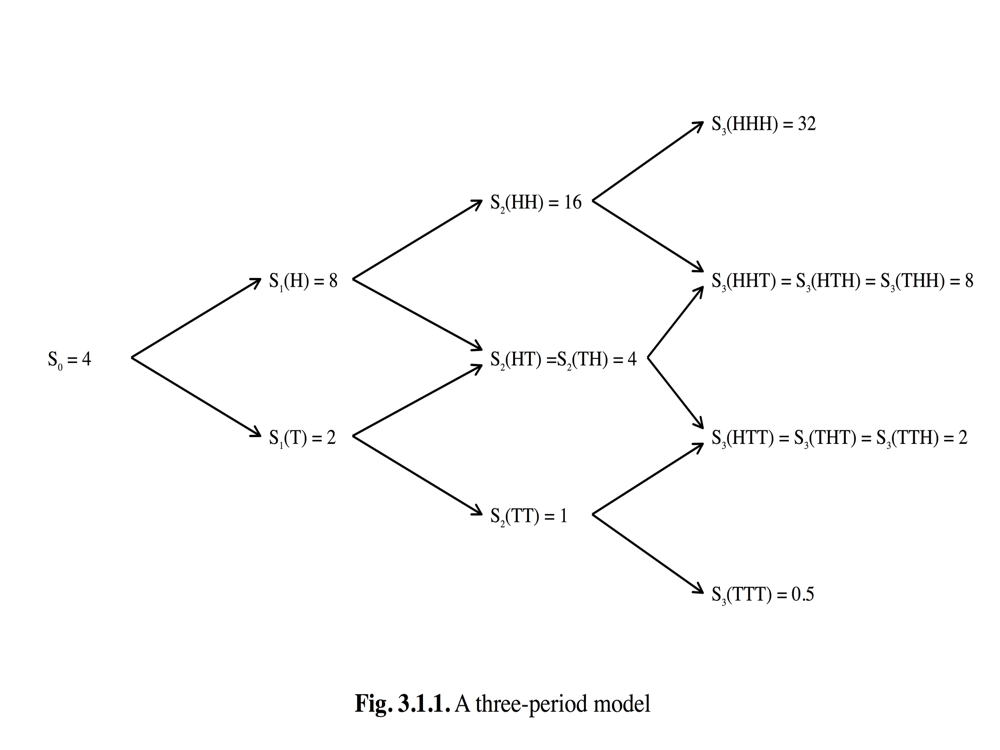【3.3】 Using the stock price model of Figure 3.1.1 and the actual probabilities $p = \frac{2}{3}, q = \frac{1}{3}$, define the estimates of $S_3$ at various times by

$$M_n = E_N[S_3]$$

Fill in the values of $M_n$ in a tree like Figure 3.1.1 Verify that $M_n, n = 0, 1, 2, 3$, is a martingale.

We note that $M_3 = S_3$. We compute $M_2$ from the formula $M_2 = E_2\left[S_2\right]$:

\begin{align} M_2(HH) & = \frac{2}{3}S_3(HHH) + \frac{1}{3}S_3(HHT) = \frac{2}{3} \cdot 32 + \frac{1}{3} \cdot 8 = 24, \\ M_2(HT) & = \frac{2}{3}S_3(HTH) + \frac{1}{3}S_3(HTT) = \frac{2}{3} \cdot 8 + \frac{1}{3} \cdot 2 = 6, \\ M_2(TH) & = \frac{2}{3}S_3(THH) + \frac{1}{3}S_3(THT) = \frac{2}{3} \cdot 8 + \frac{1}{3} \cdot 2 = 6, \\ M_2(TT) & = \frac{2}{3}S_3(THH) + \frac{1}{3}S_3(THT) = \frac{2}{3} \cdot 2 + \frac{1}{3} \cdot 0.5 = 1.5. \end{align}

We next compute $M_1$ from the formula $M_1 = E_1[S_3]$:

\begin{align} M_1(H) & = \frac{4}{9}S_3(HHH) + \frac{2}{9}S_3(HHT) + \frac{2}{9}S_3(HTH) + \frac{1}{9}S_3(HTT) \\ & = 18, \\ M_1(T) & = \frac{4}{9}S_3(THH) + \frac{2}{9}S_3(THT) + \frac{2}{9}S_3(TTH) + \frac{1}{9}S_3(TTT) \\ & = 4.50. \end{align}

Finally, we compute

\begin{align} M_0 & = E\left[S_3\right] \\ & = \frac{8}{27}S_3(HHH) + \frac{4}{27}S_3(HHT) + \frac{4}{27}S_3(HTH) + \frac{4}{27}S_3(THH) \\ & \quad + \frac{2}{27}S_3(HTT) + \frac{2}{27}S_3(THT) + \frac{2}{27}S_3(TTH) + \frac{1}{27}S_3(TTT) \\ & = 13.50. \end{align}We verify the martingale property. We have $M_2 = E_2[M_3]$ because $M_3 = S_3$ and we used the formula $M_2 = E_2[S_3]$ to compute $M_2$. We must check that $M_1 = E_1[M_2]$ and $M_0 = E_0[M_1] = E[M_1]$, which we do below:

\begin{align} E_1\left[M_2\right](H) & = \frac{2}{3}M_2(HH) + \frac{1}{3}M_2(HT) = \frac{2}{3} \cdot 24 + \frac{1}{3} \cdot 6 = 18 = M_1(H), \\ E_1\left[M_2\right](T) & = \frac{2}{3}M_2(TH) + \frac{1}{3}M_2(TT) = \frac{2}{3} \cdot 6 + \frac{1}{3} \cdot 1.5 = 4.5 = M_1(T), \\ M_0 & = \frac{2}{3}M_1(H) + \frac{1}{3}M_1(T) = \frac{2}{3} \cdot 18 + \frac{1}{3} \cdot 4.5 = 13.5 = M_0. \end{align}

### Exercise 3.4

【3.4】 This problem refers to the model of Example 3.1.2, whose $Randon-Nikod\acute{y}m$ process$Z_n$ appears in Figure 3.2.1.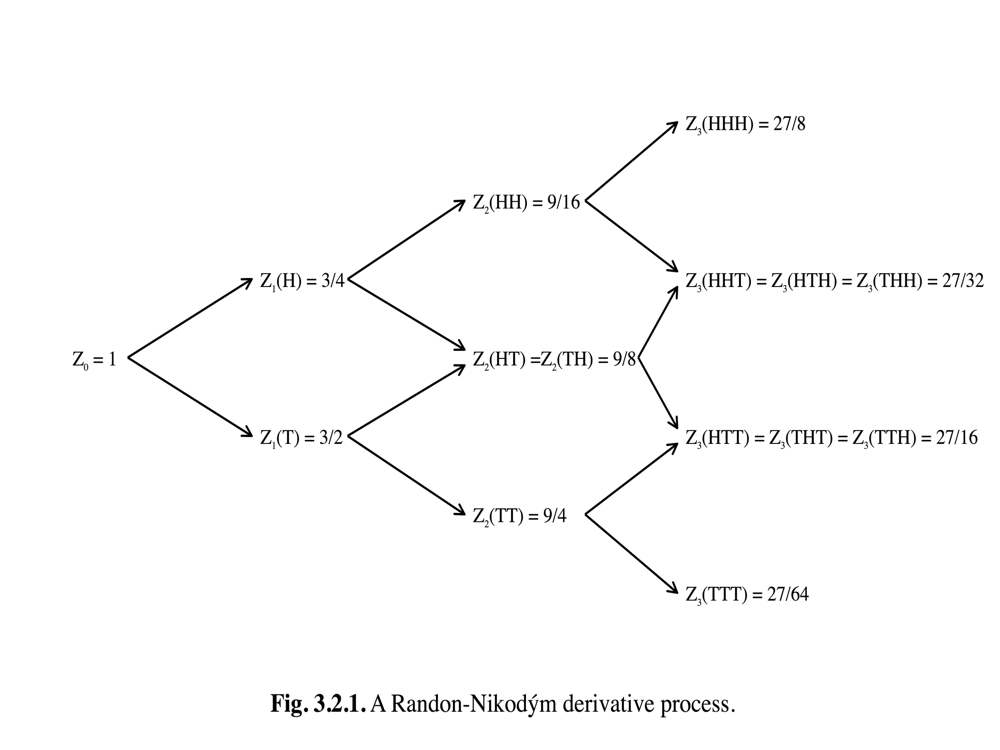(i) Compute the state price densities

\begin{align} & \zeta_3(HHH), \\ & \zeta_3(HHT) = \zeta_3(HTH) = \zeta_3(THH), \\ & \zeta_3(HTT) = \zeta_3(THT) = \zeta_3(TTH), \\ & \zeta_3(TTT) \end{align}

explicitly.

(ii) Use the numbers computed in (i) in formula (3.1.10) to find the time-zero price of the Asian option of Exercise 1.8 of Chapter 1. You should get $v_0(4,4)$ computed in part (ii) of that exercise.
(iii) Compute also the state price densities $\zeta_3(HT) = \zeta_2(TH)$.
(iv) Use the risk-neutral pricing formula (3.2.6) in the form

\begin{align} & V_2(HT) = \frac{1}{\zeta_2(HT)}E_2\left[\zeta_3V_3\right](HT), \\ & V_2(TH) = \frac{1}{\zeta_2(TH)}E_2\left[\zeta_3V_3\right](TH). \end{align}

to compute $V_2(HT)$ and $V_2(TH)$. You should get $V_2(HT) = v_2(4, 16)$ and $V_2(TH) = v_2(4,10)$, where $v_2(s, y)$ was computed in part (ii) of Exercise 1.8 of Chapter 1. Note that $V_2(HT) \not= V_2(TH)$.

In example 3.1.2:
\begin{align} V_3(HHH) & = 0, V_3(HHT) = 8, V_3(HTH) = 0, V_3(HTT) = 6, \\ V_3(THH) & = 0, V_3(THT) = 2, V_3(TTH) = 2, V_3(TTT) = 3.50. \end{align}

(i)
\begin{align} & \zeta_3(HHH) = \frac{Z(HHH)}{(1+r)^3} = 0.216, \\ & \zeta_3(HHT) = \zeta_3(HTH) = \zeta_3(THH) = \frac{Z(HHT)}{(1+r)^3} = 0.432, \\ & \zeta_3(HTT) = \zeta_3(THT) = \zeta_3(TTH) = \frac{Z(HTT)}{(1+r)^3} = 0.864, \\ & \zeta_3(TTT) = \frac{Z(TTT)}{(1+r)^3} = 1.728 \end{align}

(ii)
\begin{align} V_0 & = \sum_{\omega \in \Omega}V_3(\omega)\zeta_3(\omega)P_3\omega) \\ & = 1.216 \end{align}

(iii)
\begin{align} \zeta_2(HT) = \frac{Z(HT)}{(1+r)^2} = \frac{\frac{9}{8}}{(1+0.25)^2} = 0.72 \\ \zeta_2(TH) = \frac{Z(TH)}{(1+r)^2} = \frac{\frac{9}{8}}{(1+0.25)^2} = 0.72 \end{align}

(vi)
\begin{align} V_2(HT) &= \frac{1}{\zeta_2(HT)}E_2\left[\zeta_3V_3\right](HT) \\ & = \frac{1}{\zeta_2(HT)}[\zeta_3(HTH)V_3(HTH)p + \zeta_3(HTT)V_3(HTT)q] \\ & = \frac{1}{0.72}(0.432 \times 2 \times \frac{2}{3} + 0.864 \times 0.5 \times \frac{1}{3}) \\ & = 1 \\ V_2(TH) &= \frac{1}{\zeta_2(TH)}E_2\left[\zeta_3V_3\right](TH) \\ & = \frac{1}{\zeta_2(TH)}[\zeta_3(THH)V_3(THH)p + \zeta_3(THT)V_3(THT)q] \\ & = \frac{1}{0.72}(0.432 \times 2 \times \frac{2}{3} + 0.864 \times 0 \times \frac{1}{3}) \\ & = 0.2 \\ \end{align}

### Exercise 3.5

【3.5】 (Stochastic volatility, random interest rate). Consider the model of Exercise 2.9 of Chapter 2. Assume that the actual probability measure is

$$P(HH) = \frac{4}{9}, P(HT) = \frac{2}{9} P(TH) = \frac{2}{9} P(TT) = \frac{1}{9}.$$

The risk-neutral measure was computed in Exercise 2.9 of Chapter 2.

(i) Compute the $Randon-Niked\acute{y}m$ derivative $Z(HH), Z(HT), Z(TH), Z(TT)$ of $\tilde{P}$ with respect to $P$.

(ii) The $Randon-Nikd\acute{y}m$ derivative process $Z_0, Z_1, Z_2$ satisfies $Z_2 = Z$. Compute $Z_1(H), Z_1(T)$ and $Z_0$. Note that $Z_0 = EZ = 1$.

(iii) The version of the risk-neutral pricing formula (3.2.6) appropriate for this model, which does not use the risk-measure, is

\begin{align} V_1(H) &= \frac{1+r_0}{Z_1(H)} E_1 \left[ \frac{Z_2}{(1+r_0)(1+r_1)} \right](H) \\ &=\frac{1}{Z_1(H)(1+r_1(H))}E_1\left[Z_2V_2\right](H), \\ V_1(T) &= \frac{1+r_0}{Z_1(T)}E_1\left[ \frac{Z_2}{(1+r_0)(1+r_1)}\right](T) \\ &=\frac{1}{Z_1(T)(1+r_1(T))}E_1\left[Z_2V_2\right](T), \\ V_0 &= E\left[ \frac{Z_2}{(1+r_0)(1+r_1)}V_2 \right] \end{align}

Use this formula to compute $V_1(H), V_1(T)$, and $V_0$ when $V_2 = (S_2 - 7)^+$.
Compare the result with your answers in Exercise 2.9 (ii) of Chapter 2.

(i) In Exercise 2.9 if Chapter 2, the risk-neutral probabilities are:

$$\tilde{P}(HH) = \frac{1}{4}, \tilde{P}(HT) = \frac{1}{4}, \tilde{P}(TH) = \frac{1}{12}, \tilde{P}(TT) = \frac{5}{12}.$$

Therefore, the $Randon-Nikod\acute{y}m$ derivative is:

\begin{align} Z(HH) & = \frac{\tilde{P}(HH)}{P(HH)} = \frac{1}{4} \cdot \frac{9}{4} = \frac{9}{16}, \\ Z(HT) & = \frac{\tilde{P}(HT)}{P(HT)} = \frac{1}{4} \cdot \frac{9}{2} = \frac{9}{8}, \\ Z(TH) & = \frac{\tilde{P}(TH)}{P(TH)} = \frac{1}{12} \cdot \frac{9}{2} = \frac{3}{8}, \\ Z(TT) & = \frac{\tilde{P}(TT)}{P(TT)} = \frac{5}{12} \cdot \frac{9}{1} = \frac{15}{4}. \end{align}

(ii)

\begin{align} Z_1(H) & = E_1\left[Z_2\right](H) \\ & = Z_2(HH)P\{ \omega_2 = H | \omega_1 = H \} \\ & = Z_2(HT)P\{ \omega_2 = T | \omega_1 = H \} \\ & = Z_2(HH) \frac{P(HH)}{P(HH) + P(HT)} + Z_2(HT) \frac{P(HT)}{P(HH) + P(HT)} \\ & = \frac{9}{16} \cdot \frac{\frac{4}{9}}{\frac{4}{9} + \frac{2}{9}} + \frac{9}{8} \cdot \frac{\frac{2}{9}}{\frac{4}{9} + \frac{2}{9}} \\ & = \frac{3}{4}. \end{align}

\begin{align} Z_1(T) & = E_1\left[Z_2\right](T) \\ & = Z_2(TH)P\{ \omega_2 = H | \omega_1 = T \} = Z_2(HT)P\{ \omega_2 = T | \omega_1 = T \} \\ & = Z_2(TH) \frac{P(TH)}{P(TH) + P(TT)} + Z_2(TT) \frac{P(TT)}{P(TH) + P(TT)} \\ & = \frac{3}{8} \cdot \frac{\frac{2}{9}}{\frac{2}{9} + \frac{1}{9}} + \frac{15}{4} \cdot \frac{\frac{1}{9}}{\frac{2}{9} + \frac{1}{9}} \\ & = \frac{3}{2}. \end{align}

\begin{align} Z_0 & = E_0\left[Z_1\right] \\ & = E\left[Z_1\right] \\ & = Z_1(H)[P(HH) + P(HT)] + Z_1(T)[P(TH) + P(TT)] \\ & = \frac{3}{4} \cdot \left( \frac{4}{9} + \frac{2}{9} \right) + \frac{3}{2} \cdot \left( \frac{2}{9} + \frac{1}{9} \right) \\ & = 1. \end{align}

We may also check directly that $EZ = 1$, as follows:

\begin{align} EZ & = Z(HH)P(HH) + Z(HT)P(HT) + Z(TH)P(TH) + Z(TT)P(TT) \\ & = \frac{9}{16} \cdot \frac{4}{9} + \frac{9}{8} \cdot \frac{2}{9} + \frac{3}{8} \cdot \frac{2}{9} + \frac{15}{4} \cdot \frac{1}{9} \\ & = \frac{1}{4} + \frac{1}{4} + \frac{1}{12} + \frac{5}{12} \\ & = 1. \end{align}

(iii) We recall that

$$V_2(HH) = 5, V_2(HT) = 1, V_2(TH) = 1, V_2(TT) = 0.$$

We computed in part (ii) that:

\begin{align} P\{ \omega_2 = H | \omega_1 = H \} = \frac{\frac{4}{9}}{\frac{4}{9}+\frac{2}{9}} = \frac{2}{3}, \\ P\{ \omega_2 = T | \omega_1 = H \} = \frac{\frac{2}{9}}{\frac{4}{9}+\frac{2}{9}} = \frac{1}{3}, \\ P\{ \omega_2 = H | \omega_1 = T \} = \frac{\frac{2}{9}}{\frac{2}{9}+\frac{1}{9}} = \frac{2}{3}, \\ P\{ \omega_2 = T | \omega_1 = T \} = \frac{\frac{1}{9}}{\frac{2}{9}+\frac{1}{9}} = \frac{1}{3}, \\ \end{align}

Therefore,

\begin{align} V_1(H) & = \frac{1}{Z_1(H)(1+r_1(H))}E_1 \left[Z_2V_2 \right](H) \\ & = \left( \frac{3}{4} \cdot \frac{5}{4} \right)^{-1} [Z_2(HH)V_2(HH)P\{\omega_2 = H | \omega_1 = H\} \\ & \quad + Z_2(HT)V_2(HT)P\{\omega_2 = T | \omega_1 = H\}] \\ & = \frac{16}{15} \left[ \frac{9}{16} \cdot 5 \cdot \frac{2}{3} + \frac{9}{8} \cdot 1 \cdot \frac{1}{3} \right] \\ & = 2.40. \end{align}

\begin{align} V_1(T) & = \frac{1}{Z_1(T)(1+r_1(T))}E_1 \left[ Z_2V_2 \right](T) \\ & = \left( \frac{3}{2} \cdot \frac{3}{2} \right)^{-1} [Z_2(TH)V_2(HTH)P\{\omega_2 = H | \omega_1 = T \} \\ & \quad + Z_2(TT)V_2(TT)P\{\omega_2 = T | \omega_1 = T\}] \\ & = \frac{4}{9} \left[ \frac{3}{8} \cdot 1 \cdot \frac{2}{3} + \frac{15}{4} \cdot 0 \cdot \frac{1}{3} \right] \\ & = 0.11, \end{align}

and

\begin{align} V_0 & = E \left[ \frac{Z_2V_2}{(1+r_0)(1+r_1)} \right] \\ & = \frac{Z_2(HH)V_2(HH)}{(1+r_0)(1+r_1(H))}P(HH) + \frac{Z_2(HT)V_2(HT)}{(1+r_0)(1+r_1(H))}P(HT) \\ & \quad + \frac{Z_2(TH)V_2(TH)}{(1+r_0)(1+r_1(T))}P(TH) + \frac{Z_2(TT)V_2(TT)}{(1+r_0)(1+r_1(T))}P(TT) \\ & = 1.00444. \end{align}